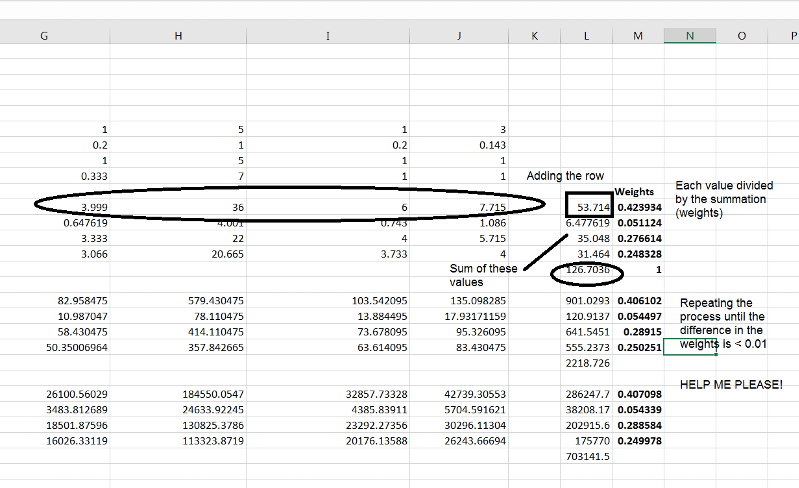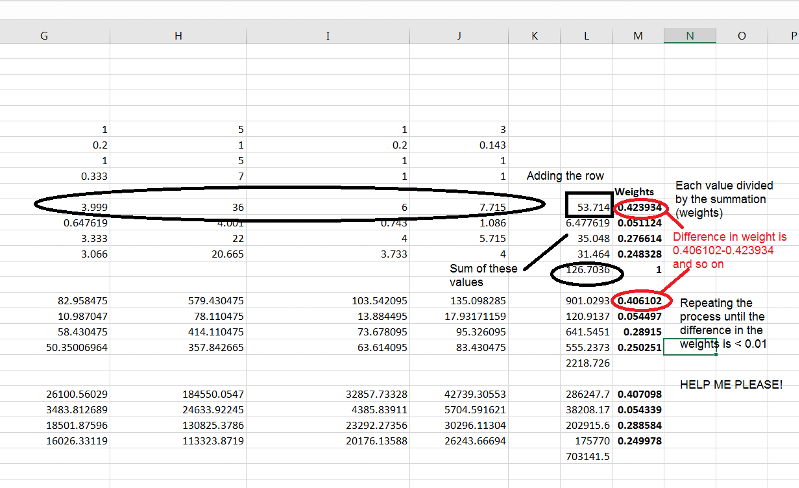Hello everyone.
I have a matrix that I want to square (multiplying it by itself).
and then I'm gonna add the value of each row of this matrix, and then add the value of this column and divide each value by the total.
Look at this picture and you will understand what I mean.I need a code to repeat this process until the difference in the weight is < 0.01 (probably using loops).

Im using this code to square the matrices:
``````  Dim xArray As Variant, zArray As Variant
Dim Fn As Object
Set Fn = Application.WorksheetFunction
xArray = Range("g11:j14").Value
zArray = Fn.MMult(xArray, xArray)

ActiveCell.Resize(4, 4).Value = zArray

``````
Who is Participating?
I wear a lot of hats...

"The solutions and answers provided on Experts Exchange have been extremely helpful to me over the last few years. I wear a lot of hats - Developer, Database Administrator, Help Desk, etc., so I know a lot of things but not a lot about one thing. Experts Exchange gives me answers from people who do know a lot about one thing, in a easy to use platform." -Todd S.

Author Commented:
The "difference in the weights" is each value of the weight minus the value of the previous weight.
Like this:Commented:
repeat until all the weight differences in two adjacent sets are < .001 or until a single comparison is < .001?
Author Commented:
Repeat until the weight differences in two adjacent sets are < 0.001
Which means
A-a < 0.001 and B-b <0.001 and C-c <0.001 and D-d<0.001
Commented:
You could set up a formula that checks that
``````=And((L15-L9)<.001,(L16-L10)<.001,(L17-L11)<.001,(L18-L12)<.001)
``````

Then, select two adjacent blocks and the checker cell and do a fill down, dragging the selection by the lower right corner until the checker value equals True.
Author Commented:
What I need is a loop that keeps squaring the matrix until the difference between the weight of the squared matrix and the weight of the previous matrix is < 0.001. That's what I mean bro.
I already know the code to square the matrix. but I dont know how to create a loop that keeps squaring the matrices.
Commented:
Try this
``````Sub Q_29010395()
Dim wks As Worksheet
Dim rngPrior As Range
Dim rngCurrent As Range
Dim rng As Range
Dim boolStop As Boolean

Dim vArray As Variant   ', zArray As Variant
Dim Fn As Object

Set Fn = Application.WorksheetFunction
Set wks = ActiveSheet
Set rngPrior = wks.Range("G6").CurrentRegion
Set rngCurrent = rngPrior.End(xlDown).Offset(3)

Do
vArray = rngPrior.Value
'zArray = Fn.MMult(xArray, xArray)

rngCurrent.Resize(4, 4).Value = Fn.MMult(vArray, vArray)
rngCurrent.CurrentRegion.Columns(4).Offset(0, 2).FormulaR1C1 = "=sum(rc[-5]:rc[-2])"
rngCurrent.CurrentRegion.Columns(4).Offset(0, 3).FormulaR1C1 = "=rc[-1]/sum(r" & rngCurrent.Row & "c[-1]:r" & rngCurrent.Row + 3 & "c[-1])"
boolStop = True
For Each rng In rngCurrent.CurrentRegion.Columns(4).Offset(0, 3).Cells
If (rng - rng.Offset(-6, 0)) < 0.001 Then
Else
boolStop = False
Exit For
End If
Next
If boolStop Then
Exit Do
Else
'set up next iteration
Set rngPrior = rngCurrent
Set rngCurrent = rngCurrent.End(xlDown).Offset(3)
Set rngPrior = rngPrior.CurrentRegion
End If
'Stop
Loop Until boolStop
End Sub
``````
Q_29010395.xlsm

Experts Exchange Solution brought to you by

Facing a tech roadblock? Get the help and guidance you need from experienced professionals who care. Ask your question anytime, anywhere, with no hassle.

Author Commented:
Author Commented:
Im using a command button in a userform
Commented:
Did you look at my workbook?

The code assumes a certain configuration of cells to start.  If your worksheet does not have that configuration, I would expect the code to fail.
Author Commented:
Oh, my bad. It's working perfectly!! thank you so much indeed!!
1 last request, please. I want to record the results of the last weights in a fixed range of cells.
I mean no matter how I change the values of the matrix. after the loop ends, I want the results of the last weights recorded in some fixed range of cells. Let's say cells (O6:O9). can you please help me? and thank you a lot!!! you are truly a life saver!
Commented:
That is a new feature, so that should be a new question.
Author Commented:
and can you plz show me what changes do I need to make to the code in case I wanted to change the matrix into 5x5?